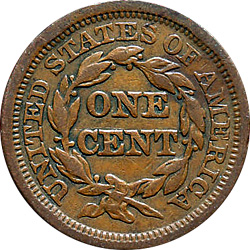# Where will the coin drop?

Probability Level 2There are 2 circles. Once circle has a radius of 40 centimeters and the other circle is inside the first circle with a radius of 20 centimeters. If I randomly drop a coin with a radius of one centimeter inside the larger circle, what is the probability that the coin will land in the smaller circle without any part of the coin touching outside the circle.

NOTE: this question is flawed as Brian in the solution discussion pointed out.

×

Problem Loading...

Note Loading...

Set Loading...